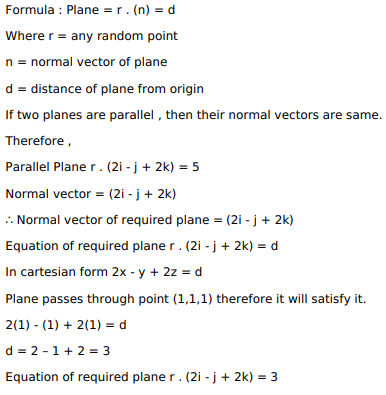# Find the vector equation of the plane passing through the point

Question:

Find the vector equation of the plane passing through the point $(1,1,1)$ and parallel to the plane $\overrightarrow{\mathrm{r}} \cdot(2 \hat{\mathrm{i}}-\hat{\mathrm{j}}+2 \hat{\mathrm{k}})=5$.

Solution: